The Rotation of the Moon

New Version Dec. 12 2012

Update for the layman and Scientists

As the spin in the community of Astrophysics has yet been able to grasp even the simplest concepts in the universe, due to holding dear to their flat earth theories, we will address why the Moon does not rotate once about its axis per one orbit about the earth. Yet as of today NASA’s spokesman astronomer Phil at still believes in this myth. Either you are a paid debunker or have little wisdom of the universe, which is it Phil?

Now lets clear away all of the smoke and mirrors in one theory of Astronomy which still holds dear today, the rotation of the Moon. For those who truly want to learn, you need to throw out all of that you have learned on this subject and let’s start on a fresh unwritten page.

We can all agree that rotation is defined as an object that spins about a central axis from a set point and returns to that same point after the rotational arc created completes a defined form a circle composed of 360 degrees as measured on earth in a perfect representation of an orbit or a variation of an ellipse as outside forces affect the orbit.

Let’s examine a single rotation for a static object as of position within the universe where a imaginary plane bisects the planetary sphere in this case is represented by its equator while the axis of rotation maintains an angle of 90 degrees of separation. The plane will now be segmented into a grid to where a point on the equator on the surface of the sphere is designated as point A, a line drawn between that point and the axis of rotation is designated as a directional point of 0 degrees. At this point, let’s assign 3 more yielding a total of 4 reference points on this sphere at the .25 point increments along motion of one rotation starting at 0 degrees then moving through 90, 180, 270 degrees and returning to point origin 0.

We now can now extend lines of reference all starting at the axis of rotation through these 4 points along the equatorial circle outwards until they pierce the orbital circle of the Moon within the same plane. We have now set up a grid plane that has reference points in relation to static points of quarter separation on the surface of the earth and its axis and their reference intersection points with the path of the orbit of the Moon.

The next step you need to examine is the simplistic motion of a sphere’s axis moving in a straight line while factoring in a single rotation of 360 degrees about this same line. The timeline of one rotation of the sphere is going to be set to a particular but static length along the path. Where the word "length" is designated to the distance of the sphere travels along the path.

Now let’s establish a set of equi-distant reference points along this path where the total distance is equal to a particular length which is static or does not change for this example. The initial direction of the line will be pointed to the west. The point of 0 degrees is set to what then expands in the direction of what you call west only on a designed grid unrelated to the earth’s magnetic field, but a paper reference, as is the point of origin. At .25 of distance of length, a clockwise rotation of the sphere is aligned with the path of direction now shifts to 90 degrees away from the path. At .5 of length the point of rotation of the sphere is directed at 180 degrees from the path or east pointing towards the rear of movement along the path. And at .75 of length again along its path, the sphere’s point of reference on its surface is pointed south at 270 degrees. At the completion of motion, the end of path of a static distance of length, rotation finds us completing the full 360 degrees or one rotation about the axis of the sphere. Visualizing the equator on its edge from above the axis reveals a completed circle. Thus we have returned to a point 0 degrees or the point of origin looking west on the reference grid. So we are now aware of how rotation of a sphere behaves when in motion along a straight line with a defined distance along a path which is equal to a static length where the passage of time for the sphere to move from the point of origin and back equals the period of one rotation. So do all of you follow me so far? If not, return to the top of the page until you understand the concept before moving forward.

The next step is to consider the concept of no rotation of and spherical object with an established point of reference which is directed west when moving in a straight line. All here can agree with no rotation while in motion along a straight line where the reference distance is still the established static length. You observe at all points along the path the point on the equator of the sphere without rotation remains pointed west. This will be the counter reference line addressing no rotation about the axis of the sphere while move along distance of length.

Now let’s address motion within the plane of rotation occurring along a straight path when it is affected by an outside force. All of you as layman and scientists are familiar with the current definition of orbital motion of a moon or object about a planet or large mass. The path of its determinate orbit is independent of its internal motion, but is modified by the flow of gravity towards the planet affecting the object thus creating what is called a satellite orbit as its path or direction that curves towards to planet. The point if the force of gravity counters the centrifugal force outward a stable orbit is form is the eyes of human scientists. This concept is wrong, but will not be addressed here for this discussion. So the human concept of an orbit will be used in this example.

Mankind needs to differentiate between the concept of a curved path and rotation at a spherical axis along that path when addressing the concept of the rotation of the Moon.

Now for the final comparison, let the path of a distance of "length" be replaced by the distance the Moon travels in its circular orbital path about the earth. Segment this same circular path affected by gravity to be a static equal to the distance the Moon travels in one orbit. So every 90 degrees traveled along the circular orbit by lines using the axis of the earth and point of intersection of the orbital path to set up standard reference points, now what was a straight line when affected by gravity is a curved line that forms an orbit. Follow me so far? If not start at the top again until you understand.

You when you use the path with rotation as the line which curves due to gravity, rotation, still occurs about the line with the initial point of west pointed inward towards earth. The various reference points and positions do not reflect the same reference point directed towards the surface of the earth or west. As they now point out into space of various locations as a determinate of position along the path.

When you examine the counter reference line of no rotation where the western look is pointed always towards the planet and the path of the line is curved towards earth by gravity, it is always visible. When spherical rotation occurs along a curved orbital path at the 90 degree reference point we see a different surface of the orbital satellite. When there is no rotation of a sphere in orbit about a planet or mass the same surface or face is seen always as gravity is responsible for the change of direction and the same face, not one rotation synchronized with one orbital period.

As scientists and some laymen, your lab reports will be to recreate the concept by using a flexible line to simulate the path of length and spot a sphere at .25 increments while shifting rotation 90 degrees per segment. Then curve the line as a response to gravity using both scenarios. What do you see? Do your results conform to established theories? It is time to move on. Throw out all that you have been taught and start from scratch. So how do you handle the rebuttals? Ask them how does their model represent the motion of the Moon if there was an absent of rotation about the axis of he Moon. For many encounters their answer will be silence or I will get back to you.

Old Version

The Brief: Today’s astronomers explain the Moon’s rotation about its axis with one side visible to Earth. The theory states, the moon spins about its axis in synchronization with its orbital period around the Earth. This revolution or slow spin of the axis of the Moon allegedly turns precisely at a rate, which keeps the same side always facing the Earth. We examine this theory to address the truth behind this hypothesis or present a new solution.

The current views and theories states the Moon rotates once about its axis for every orbit around the Earth. Here is a quote from a well respected astronomy website "Bad Astronomy"  that won the 2004 Scientific American science & technology web award.

 "How it works: If you go out on several different nights and look at the Moon, you will always see the same features, at about the same position. It looks as if the Moon doesn't rotate! Ah, but it does. This can be seen using a model. Grab two oranges (or apples, or baseballs, or whatever roughly spherical objects you have handy). Mark one with an "X"; this represents a feature on the Moon. Now put the other one down on a table; this is the Earth. Place the Moon model on the table about 30 centimeters (one foot) away with the X facing the Earth model. Now move the Moon model as if it were orbiting the Earth, taking care to make sure that the X faces the Earth model at all times. Surprise! You'll see that to keep the X facing the Earth model, you have to rotate the Moon model as it goes around the Earth model. Furthermore, you can see you have to spin it exactly once every orbit to keep the X facing the Earth model. If you don't rotate it, the Moon model will show all of its "sides" to the Earth model as it goes around. Now, I have been a bit tricky here. We are talking about two different frames of reference; one on the surface of the Earth looking out at the Moon, and one outside the Earth-Moon system looking in. You performed the experiment from the latter frame, and saw the Moon rotating. From the former, however, you can see for yourself the Moon does not rotate. What is being argued here is that in one frame the Moon rotates, in another it does not. We've actually learned three things: # 1) the Moon rotates as it orbits the Earth (as seen by an outside observer); # 2) it rotates one time for every orbit (to that observer); and # 3) if it didn't rotate, we would eventually see all of the Moon as it orbited the Earth. " Courtesy of *Bad Astronomy http://www.badastronomy.com/bad/misc/moon_spin.html

*This website does not endorse or agree with anything written in the Grant Chronicles, but has in the past provided  strong counter views on its forums from its members.

Now advance 4 years to 2008 and how views have changed, the Discovery Channel has done a documentary on extra solar planets and presented a theoretical planet locked by gravity where rotation of the planet does not occur. In this example, the star and the planet in a mutual orbit presents the same face of the planet. How does this occur with the current theories of Moon rotation?

Let's examine the current status quo for Moon rotation

Currently in 2011, the status quo within the field of Astronomy is that the Moon spins about its axis in a period equal to its approximate 27.322 day rotational period around Earth. So lets looks at the frame of reference used in current theories within the field of Astronomy that has backed this conclusion. When scientists concluded the axis is the Moon rotated in pace with its orbit presenting the same side always, this seemed true, but it was the frame of reference used, which is the source of confusion.

Lets go over a few of mankind's facts pertaining to motion. An orbit is where gravity curves a path of an object to revolve about a central point. The definition  rotation considers the point of reference used, if the object rotating about a point of reference outside of the object this motion is an orbit. If the rotation is a reference point within the object, this is rotational spin.

Astronomers have made a basic mistake in orbital mechanics and when given the answer refuse consider that they are wrong. It has been a decade since some of you in the field have seen this paper and debated it on the forums, yet "Ask the Astronomer" still has not learned. The reference frame used included the Earth as the central point, yes a point on the Moon's surface rotates, but this is due to gravity curving the path of the Moon. The Moon does not rotate 360 degrees about its internal axis as rotational spin. Within in this frame of reference, the Moon's axis follows its rotational path as gravity turns the direction of motion of the Moon curving inward, but maintaining orbital distance.  Astronomers again when giving a simple explanation involving the motion of the Moon contradict their own words describing orbital rotation and spin.  The problem with the current theories on Moon rotation is that those who formulated this theory, confused completing a curved path of rotation around the Earth as the Moon was slowly turning with reference points of the Moon changing in relation to the planet. It is only the illusion of rotation as others in an expanded reference frame can revolve an object about a point and you seem to be turning it. The key here is turning it not spinning about its axis. This is simple orbital mechanics 101.

For the layman let examine a simple example a sphere will circle in the air about the point you standing. The painted side revolves faces you always. Now we replace the sphere with a helicopter it circles and we see the same side, but speeds up and slows and we still see the same side. Now observe the rotor if it is off the same blade faces us. Now let that rotor spin once about the axis of the helicopter with the closest blade marked. Once the helicopter made it half way around you find the marked blade on the far side of the copter only to show itself on the inside on completion of the circle. Only from above looking down do you see the copter heading first south, then turning west then north then east simulating rotation, but it is only a change in direction. You also saw that orbital velocity had nothing to do with seeing the same side due to synchronization of the rotational period and its spin about its axis for the Moon.

A Simplistic Model

A Description of Moon Rotation about its Axis

The first assumption that has to be dropped is the confusion over frame of reference. If the Moon was a railroad car and its orbital path is determined by tracks, we realize the car is always turning towards the Earth. This is due to gravity constantly bending the path of the Moon towards resulting in an orbit being created. There is a different between a gravity induced curve path or orbit and rotational spin about an axis. The two are inherently different as the point of reference related to rotation moves from the axis within the Moon to the Earth. Ask yourself does the car turn while driving a complete circle or complete one spin about its center during the same trip around the circle?

Lets consider a fresh approach to solving this problem.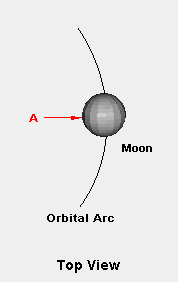In the lateral diagrams A is the initial reference point and will be assigned a location of zero degrees with measurements proceeding 360 degrees counter-clockwise back to that point.

Diagram 1

In Diagram 1 we have setup the orbital path of the Moon around the Earth and designated the middle of the face of the Moon, which we see on most nights as reference point A for the extreme eastern part of the orbit. The Moon location in Earth orbit and point A will have the same initial value of 0 degrees and additional reference points will rotate counter-clockwise.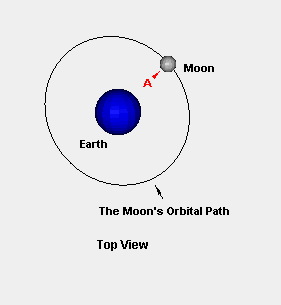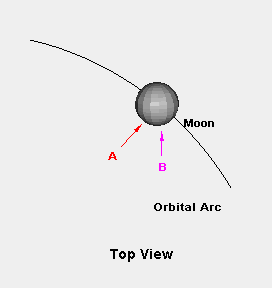In the lateral diagrams A is still the initial reference point on the face of the Moon, but has moved from initial point @ 0o along the orbital path to point B is located @ 45o in a counter-clockwise orbital direction.

Diagram 2

In diagram 2 we begin to perceive the idea of rotational spin about the axis of the Moon while in orbital motion about the Earth. Here we have moved the position of the moon 45 degrees counter-clockwise along its orbital path. Point B represents a 45 degree movement of the axis in relation to the tangent line of the orbital path. Remember the motion or curved path is due to gravity affecting forward motion of the Moon by turning not rotating.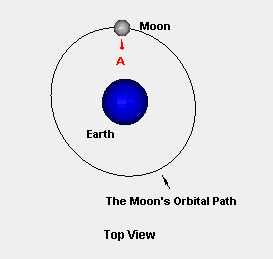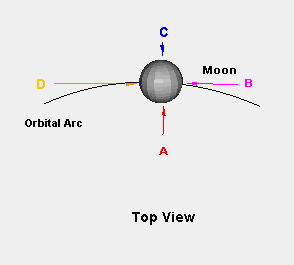In the lateral diagrams A is still the initial reference point @ 0o to the surface of the Earth, point B is located @ 90o, point C is located @ 180o and point D is located @ 270o counter-clockwise along the Moon’s circumference.

Diagram 3

Finally here in Diagram 3 lets examine the designated points and equate their position to rotation about the Moon's axis in relation to one orbit. If point A reached point D @ 270 degrees in a 90 degree sweep or a quarter orbit, the Moon would spin about its axis 3 times at the completion of one orbit. If point A reached point C @ 180 degrees in a 90 degree sweep or a quarter orbit, the Moon would spin about its axis 2 times at the completion of one orbit. The key to math is continuity. Now if point A reached point B @ 90 degrees in a 90 degree sweep or a quarter orbit, the Moon would spin about its axis 1 time at the completion of one orbit. Point A is a point where no rotation about an axis occurs. Again point A maintains a perpendicular orientation to the closest Earth tangent line along the Moon's orbital path.

Explanation of Frames of Reference

Astronomers observing the Moon noticed that it seems to be rotating on its axis in precise synchronization with its orbital speed, but their conclusion is so wrong. A close examination would reveal the Moon does not rotate at all and is void of spin. If the face of the Moon was like a tire and this tire turned one time to complete an orbit. Does not the surface of tire move as it revolves? You did say the Moon rotates about its axis, so how can the same point on a tire be seen if it rotates once about its axis from a reference point inside the orbit?

Here is an experiment that can be conducted in your elementary astronomy labs. Reviewing an object rotates when the reference point that spin occurs is within the object itself. A set up a simple clear rigid plastic 16 inch square sheet, 1/2 inch thick. Drill a hole in its center and attach a rigid rod. Drill a hole through center of a 2 inch wafer the same diameter as the rod, glue several inches above the base to the rod. This will be a base. Drill just greater than the rod diameter through the center of a six inch or its closest centimeter equivalent in diameter hard plastic hollow sphere. At its equator, drill another hole 90 degrees to the central axis. Glue or epoxy a hard straw at to opposite attach a 2 inch Styrofoam ball glue in place.

Rotational spin within the object is defined as the axis line (a straight line drawn from pole to pole) moving away from the reference point and returning to while moving in one direction. In the absence of rotation, the points of the axis line and the initial reference point remain aligned. Now that a rigid rod is attached to the Styrofoam ball (the Moon) and the six inch plastic sphere (Earth) we expand our frame of reference. Spinning the Earth causes the Moon to orbit as the rod represents gravity that turns the path of the Moon into a curved path and at the completion of one revolution an orbit. Upon observation the same face of the Styrofoam ball representing the Moon is always pointed toward the plastic representation of Earth no matter what the orbital velocity that occurs. Again as you notice the sphere representing the Moon does not spin about the axis within, you constantly see the reference point and the axis line are locked. If it did spin you would have to see all sides of the sphere from Earth. Looking at the total picture we find that a sphere with no rotation when pivoting about a point outside of the sphere presents the same view of the reference point and axis line. In the second part when the speed of the rotation of the sphere to completes 1 rotation within the grid was matched to pivoting about 360 degrees in synchronization you still see all sides. Now realize the Moon is the sphere within the grid and the pivot point is Earth not the Moon axis and this change the view of mankind's current observations?  Can astronomers present a simple experiment with models that backs the how Moon rotates? No. Now we should finally move on.

Now Questions?

If the Moon does not rotate, can you present a new concept of what is responsible for planetary or stellar rotation?

A simple illustration of what is responsible for rotation of a cosmic object with a fluid inner core can be shown with an egg example, not that an egg has anything to do with spin about an axis, but it will demonstrate the basic principle. In the universe, movement is about rotation being driven by the rotation frictional force from the inner core driving the shell or crust, not because of compression of matter invoking conservation of energy thus increasing rotational spin about the axis. Although this concept of rotational spin is true during formation of the object just after a localized big bang, but over time movement stabilizes the shell, its liquid interior is still subject inequalities of the neighboring gravitational and magnetic influences. This constant attraction, resultant spin within the molten core, is perpetuated by overshooting the point of attraction, drifting until attracted again and the cycle begins again is the basis for fluid inner core rotation. The fluid core of a planet is affected by universal gravitational and magnetic forces, as there is an attempt for matter in different parts of the core to seek equalization resulting in spin. The rotating core has a frictional coefficient where its torque a product of rotational spin and mass, the surface area of the core. It is this contact that drives the dependent mass of the shell or crust, which translates into stellar or planetary surface rotational periods.

For a simple experiment, spin an egg on a smooth surface to represent the forces affecting planetary cores. Stop it for a fractional second, then let the egg go. The core, which is spinning will drag the shell, thus rotation or in the case of a planet, its crust. This is rudimentary example explaining the principles of rotation in planetary and stellar objects.

Change comes about with a fresh start.